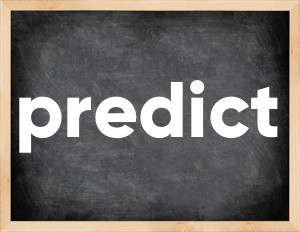# Predict past tenseEnglish verb predict [prɪˈdɪkt].
Relate to: regular verbs.
3 forms of verb predict: Infinitive (predict), Past Simple - (predicted), Past Participle - (predicted).

## Forms of verb predict in past tenses

👉 Forms of verb predict in future and past simple and past participle.
❓ What is the past tense of predict.

## Three forms of verb predict

Base Form Past Simple Past Participle
predict [prɪˈdɪkt]

predicted [prɪˈdɪktɪd]

predicted [prɪˈdɪktɪd]

## What is 2nd and 3rd form of predict?

🎓 Base Form (Infinitive) predict in Past Simple, Future Simple, Present Perfect, Past Perfect, Future Perfect?

• First form (V1) - predict. (Present simple, Future Simple)
• Second form (V2) - predicted. (Past simple)
• Third form (V3) - predicted. (Present perfect, Past perfect)

## What are the past tense and past participle of predict?

The past tense of predict is: predict in past simple is predicted. and past participle is predicted.

### What is the past tense of predict?

The past tense of predict is predicted.
The past participle of predict is predicted.

### Verb Tenses

Past simple — predict in past simple predicted. (V2)
Future simple — predict in future simple predict. (will + V1)
Present Perfect — predict in present perfect tense predicted. (have\has + V3)
Past Perfect — predict in past perfect tense predicted. (had + V3)

### predict regular or irregular verb?

👉 Is predict an irregular verb?? predict is regular verb.

## Examples of Verb predict in Sentences

•   They cannot predict the behavior of these animals. (Present Simple)
•   Oracle predicted a special mission for us (Past Simple)
•   She looked up at the sky and predicted that it would rain soon. (Past Simple)
•   Market analysts predicted a sharp drop in prices. (Past Simple)
•   The psychic has predicted a happy future for her. (Present Perfect)
•   Although many predicted that the store would go bankrupt, things are doing very well there. (Past Simple)
•   He predicted an economic crisis. (Past Simple)
•   He predicted their response with amazing foresight. (Past Simple)
•   The final result of this experiment will be impossible to predict. (Future Simple)
•   He predicted a great success for the film. (Past Simple)

Along with predict, words are popular thread and get by.

Verbs by letter: , , , , , , , , , , , , , , , , , , , , , , , , .